# Boltzmann equation

(diff) ← Older revision | Latest revision (diff) | Newer revision → (diff)

An equation in kinetic gas theory, proposed by L. Boltzmann for the determination of the single-particle distribution function of an ideal mono-atomic gas . In dimensionless variables the equation has the form: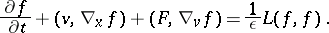(*)

Here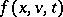is the density of the distribution function of the number of particles in the phase space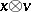,is the three-dimensional space coordinate,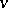is the velocity,is the time,is the field strength of the external forces, andis a dimensionless parameter (which is proportional to the ratio between the average distance covered by the particles between successive collisions and the typical scale of the phenomena under consideration). In the simplest case the collision operator has the form: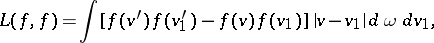where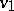andare the velocities of the molecules before collision,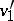and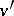are the velocities of the molecules after collision, andis the solid angle element in the direction of the vector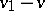.

In deriving the Boltzmann equation it is assumed that the evolution of the functionis determined by its value at a given moment of timeand by the pairwise collisions between the gas molecules, and that the time of interaction between two gas molecules during collision is much shorter than the time during which they move independently of each other. From the mathematical point of view the derivation of the Boltzmann equation is based on a certain algorithm which constructs the operator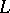in accordance with the well-known laws of motion of two gas molecules which collide with one another.

In equation (*) the range of variation of the variableis the half-line; the range of variation ofis the entire space; and the range of variation ofis a subspacein(may coincide with). In accordance with its physical meaning, the functionshould be non-negative and such thatThe simplest boundary condition on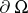has the form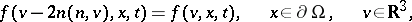whereis the normal to. There exist a number of rigorous statements of the Cauchy problem for equation (*).

How to Cite This Entry:
Boltzmann equation. Encyclopedia of Mathematics. URL: http://encyclopediaofmath.org/index.php?title=Boltzmann_equation&oldid=12483
This article was adapted from an original article by A.A. Arsen'ev (originator), which appeared in Encyclopedia of Mathematics - ISBN 1402006098. See original article